Courses

# Olympiad Test: Patterns - 2

## 20 Questions MCQ Test Science Olympiad for Class 5 | Olympiad Test: Patterns - 2

Description
This mock test of Olympiad Test: Patterns - 2 for Class 5 helps you for every Class 5 entrance exam. This contains 20 Multiple Choice Questions for Class 5 Olympiad Test: Patterns - 2 (mcq) to study with solutions a complete question bank. The solved questions answers in this Olympiad Test: Patterns - 2 quiz give you a good mix of easy questions and tough questions. Class 5 students definitely take this Olympiad Test: Patterns - 2 exercise for a better result in the exam. You can find other Olympiad Test: Patterns - 2 extra questions, long questions & short questions for Class 5 on EduRev as well by searching above.
QUESTION: 1

### Select a figure from the options below which will continue the series established in the five problem figures.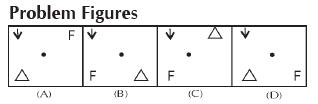Solution:

Triangle changes its position from
centre to right hand bottom corner
and the arrow moves 90° clock
wise at the same position i.e. Left
hand top corner F will come after E.

QUESTION: 2

### Find the next figure in pattern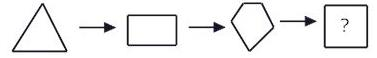Solution:

in the series, 6 sided polygon will be the next

QUESTION: 3

### find out the missing numbers /letters / figures from the given responses.

Solution: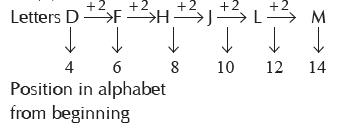QUESTION: 4

find out the missing numbers /letters / figures from the given responses.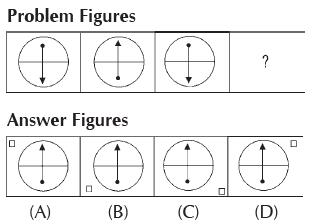Solution:

The position of small square moves
in the pattern of +1, +2, +3. So,
after leaving two corners. Position
(D) in answer figure is achieved.

QUESTION: 5

find out the missing numbers /letters / figures from the given responses.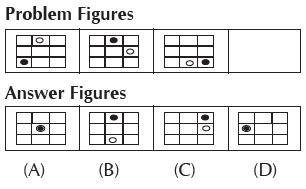Solution:

In problem figure black dot moves three steps in clockwise direction and white dot moves two steps in clockwise direction.

QUESTION: 6

find out the missing numbers /letters / figures from the given responses.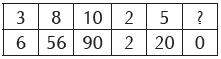Solution: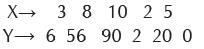1st Column – 32–3 = 9–3 = 6
2nd Column – 82–8 = 64 –8 = 56
So, pattern followed is X2 –X = Y
So 12 –1 = 0

QUESTION: 7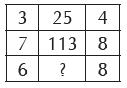Solution:

If we consider these columns as X,
Y and Z, then we get the pattern

X2 + Z2 = Y
32 + 42 = 9 + 16 = 25
72 + 82 = 49 + 64 = 113
62 + 82 = 36 + 64 = 100

QUESTION: 8

Find out the Wrong term in the series.

3, 10, 27, 4,16, 64, 5, 25,125

Solution:

The pattern followed is 3, 32, 33, 4,
42, 43, 5, 52, 53.
So, 10 should be replaced by 9.

QUESTION: 9

Find out the Wrong term in the series.

5, 10, 17, 24, 37

Solution:

The pattern followed is +5,+7,+9,+11
5 + 5 = 10
10 + 7 = 17
17 + 9 = 26
26 + 11 = 37

QUESTION: 10

Find out the Wrong term in the series.

20, 40, 200, 400, 2000, 4000, 8000

Solution: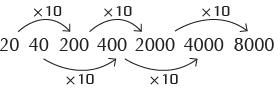So, 20,000 will replace 8000.

QUESTION: 11

How many circles will be there in pattern 10.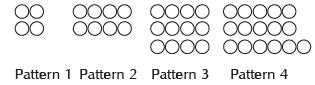Solution:

The pattern followed is 4×N
where N = no. of pattern

no. of circles in pattern 1
= 4×1 = 4
no. of circles in pattern 2
= 4×2 = 8
So the number of circles in pattern
= 4×10 = 40

QUESTION: 12

Select the figure from answer figures which completes the pattern.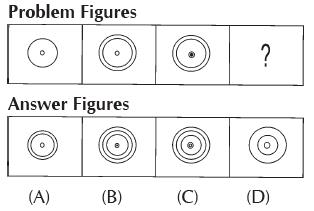Solution:

In each successive figure one circle increases at outer and inner respectively.

QUESTION: 13

Find the missing number: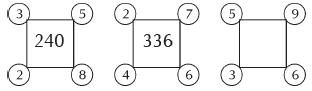Solution:

Number inside the square is multiplication of numbers present on corners.
3×5×8×2 = 240
2×7×6×4 = 336
Similarly, 5×9 ×6 ×3 = 810

QUESTION: 14

Select the figure from answer figures which completes the pattern.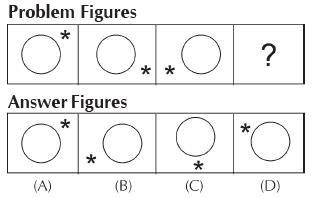Solution:

Star in each successive figure
moves one step clockwise.

QUESTION: 15

Select the figure from answer figures
which completes the pattern.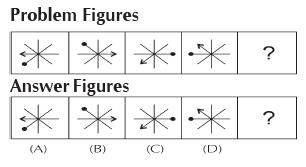Solution:

In the problem figures the arrow moves 4, 3, 2 and 1 step respectively in clockwise direction and black dot moves 2, 3, 4 and 5 steps respectively in clockwise direction.

QUESTION: 16

In the following series, what will be the 8th term?
2, 6, 18, 54, ______________

Solution:

The series follows the following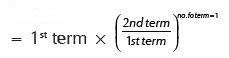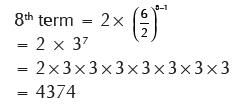QUESTION: 17

Find out the wrong term in the following series.
2, 3, 9, 27, 112, 565, 3396

Solution:

The series follows the following
pattern
2×1+1 = 3
3×2 + 2 = 8
8 × 3 + 3 = 27
So, the number 9 is wrong and
should be replaced by 8.

QUESTION: 18

Study the matrix below that follows a certain rule row-wise or column-wise. Find the missing number.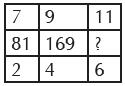Solution:

In the given matrix following pattern
exits.
Centre row = (Top row + bottom
row)2
81 = (7 + 2)2 = 92
169 = (9+4)2 = 132
In the same way (11 + 6)2 = 172 =
289

QUESTION: 19

See the figure blow and tell how many match sticks are needed to male pattern 5?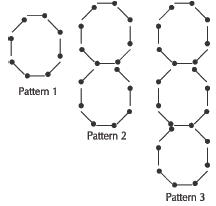Solution:

In Pattern 1, number of matchsticks
= 8 + 7(1–1)
In pattern 2, number of matchsticks
= 15 = 8+7(2–1)
In pattern 3, number of matchsticks
= 22 =8+7(3–1)
So, in pattern 5, number of
matchsticks = 8+7×(5–1)
= 8 + 7 × 4
= 8 + 28
= 36

QUESTION: 20

Select a figure from the answer figures which completes the pattern of problem figure (X)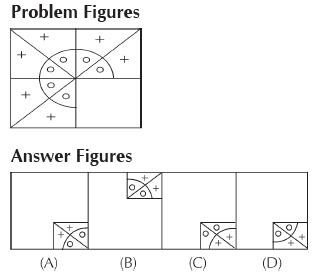Solution:

Figure (D) completes the problem
figure (X)